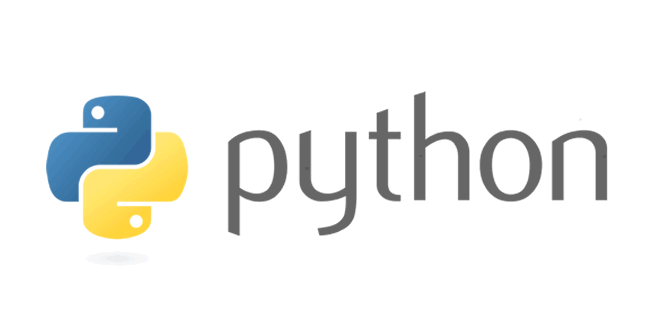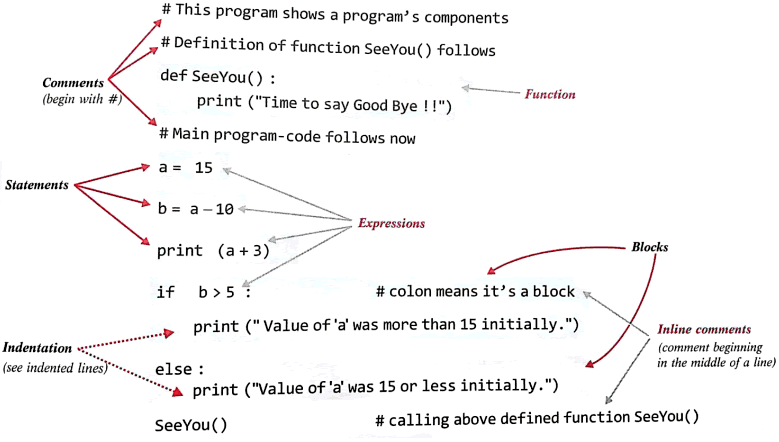Thursday , June 30 2022# Python Fundamentals: 11th Computer Science Chapter 02

 Chapter Name: Python Fundamentals [Chapter 02] Class: 11th Subject: Computer Science

### 2.1 Python Character Set: Ch 02 – Python Fundamentals

It is a set of valid characters that a language recognize.

• Letters: A – Z, a – z
• Digits: 0-9
• Special Symbols
• Whitespace

### 2.2 Tokens

Token: Smallest individual unit in a program is known as token.

There are five types of token in python:

1. Keyword
2. Identifier
3. Literal
4. Operators
5. Punctuators

### 01. Keyword

1. Keyword: Reserved words in the library of a language. There are 33 keywords in python.

All the keywords are in lowercase except 03 keywords (True, False, None).

### 02. Identifier

2. Identifier: The name given by the user to the entities like variable name, class-name, function-name etc.

#### Rules for identifiers:

• It can be a combination of letters in lowercase (a to z) or uppercase (A to Z) or digits (0 to 9) or an underscore.
• Keywords cannot be used as an identifier.
• We cannot use special symbols like !, @, #, \$, %, + etc. in identifier.
• _ (underscore) can be used in identifier.
• Commas or blank spaces are not allowed within an identifier.

### 03. Literal

3. Literal: Literals are the constant value. Literals can be defined as a data that is given in a variable or constant.

#### A. Numeric literals:

Numeric Literals are immutable.

Example: 5, 6.7, 6+9j

#### B. String literals:

String literals can be formed by enclosing a text in the quotes. We can use both single as well as double quotes for a String.

Example: “Aman” , ‘12345’

#### Escape sequence characters:

 \\ Backslash \’ Single quote \” Double quote \a ASCII Bell \b Backspace \f ASCII Formfeed \n New line character \t Horizontal tab

#### C. Boolean literal:

A Boolean literal can have any of the two values: True or False.

#### D. Special literals:

Python contains one special literal i.e. None.

None is used to specify to that field that is not created. It is also used for end of lists in Python.

#### E. Literal Collections:

Collections such as tuples, lists and Dictionary are used in Python.

### 04. Operators

4. Operators: An operator performs the operation on operands. Basically there are two types of operators in python according to number of operands:

A. Unary Operator
B. Binary Operator

A. Unary Operator: Performs the operation on one operand.

Example:

 + Unary plus – Unary minus ~ Bitwise complement not Logical negation

B. Binary Operator: Performs operation on two operands.

### 05. Separator or Punctuator

5. Separator or punctuator: , ; , ( ), { }, [ ]

### 2.3 Mantissa and Exponent Form:

A real number in exponent form has two parts:

• Mantissa
• Exponent

Mantissa: It must be either an integer or a proper real constant.
Exponent: It must be an integer. Represented by a letter E or e followed by integer value.

 Valid Exponent form Invalid Exponent form 123E05 1.23E07 0.123E08 123.0E08 123E+8 1230E04 -0.123E-3 163.E4 .34E-2 4.E3 2.3E (No digit specified for exponent) 0.24E3.2 (Exponent cannot have fractional part) 23,455E03 (No comma allowed)

### 2.4 Basic terms of a Python Programs: Ch 02 – Python Fundamentals

A. Blocks and Indentation
B. Statements
C. Expressions

#### A. Blocks and Indentation:

• Python provides no braces to indicate blocks of code for class and function definition or flow control.
• Maximum line length should be maximum 79 characters.
• Blocks of code are denoted by line indentation, which is rigidly enforced.
• The number of spaces in the indentation is variable, but all statements within the block must be indented the same amount.

For example:

if True:

print(“True”)

else:

print(“False”)

#### B. Statements:

A line which has the instructions or expressions.

#### C. Expressions:

A legal combination of symbols and values that produce a result. Generally it produces a value.

Comments are not executed. Comments explain a program and make a program understandable and readable. All characters after the # and up to the end of the physical line are part of the comment and the Python interpreter ignores them.

There are two types of comments in python:

• i. Single line comment
• ii. Multi-line comment

i. Single line comment: This type of comments start in a line and when a line ends, it is automatically ends. Single line comment starts with # symbol.

Example: if a > b: # Relational operator compare two values

ii. Multi-Line comment: Multi-line comments can be written in more than one lines. Triple quoted ‘ ’ ’ or “ ” ”) multi-line comments may be used in python. It is also known as docstring.Multiple Statements on a Single Line:

The semicolon ( ; ) allows multiple statements on the single line given that neither statement starts a new code block.

Example:

x=5; print(“Value =” x)

### 2.5 Variable / Label in Python: Ch 02 – Python Fundamentals

Definition: Named location that refers to a value and whose value can be used and processed during program execution.

Variables in python do not have fixed locations. The location they refer to changes every time their values change.

#### Creating a variable:

A variable is created the moment you first assign a value to it.

Example:

x = 5
y = “hello”

Variables do not need to be declared with any particular type and can even change type after they have been set. It is known as dynamic Typing.

x = 4 # x is of type int
x = “python” # x is now of type str
print(x)

#### Rules for Python variables:

• A variable name must start with a letter or the underscore character
• A variable name can only contain alpha-numeric characters and underscore (A – z, 0 – 9 and _ )
• Variable names are case-sensitive (age, Age and AGE are three different variables)

Python allows assign a single value to multiple variables.

Example: x = y = z = 5

You can also assign multiple values to multiple variables.

For example:

x , y , z = 4, 5, “python”

4 is assigned to x, 5 is assigned to y and string “python” assigned to variable z respectively.

x=12
y=14
x,y = y,x

print(x,y)

Now the result will be

14    12

#### Lvalue and Rvalue:

An expression has two values. Lvalue and Rvalue.

Lvalue: the LHS part of the expression

Rvalue: the RHS part of the expression

Python first evaluates the RHS expression and then assigns to LHS.

Example:

p, q, r= 5, 10, 7
q, r, p = p+1, q+2, r-1
print (p,q,r)

Now the result will be:

6   6   12

Note: Expressions separated with commas are evaluated from left to right and assigned in same order.

• If you want to know the type of variable, you can use type( ) function:

Syntax:

type (variable-name)

Example:

x=6
type(x)

The result will be:

<class ‘int’>

• If you want to know the memory address or location of the object, you can use id( ) function.
Example:
>>>id(5)
1561184448
>>>b=5
>>>id(b)
1561184448

You can delete single or multiple variables by using del statement.

Example:

del x
del y, z

### 2.6 Input from a user: Ch 02 – Python Fundamentals

input( ) method is used to take input from the user.

Example:

x = input( )
print(“Hello, ” + x)

• input( ) function always returns a value of string type.

### 2.7 Type Casting: Ch 02 – Python Fundamentals

To convert one data type into another data type.

Casting in python is therefore done using constructor functions:

• int( ) – constructs an integer number from an integer literal, a float literal or a string literal.
Example:
x = int(1) # x will be 1
y = int(2.8) # y will be 2
z = int(“3”) # z will be 3
• float( ) – constructs a float number from an integer literal, a float literal or a string literal.
Example:
x = float(1) # x will be 1.0
y = float(2.8) # y will be 2.8
z = float(“3”) # z will be 3.0
w = float(“4.2”) # w will be 4.2
• str( ) – constructs a string from a wide variety of data types, including strings, integer literals and float literals.
Example:
x = str(“s1”) # x will be ‘s1’
y = str(2) # y will be ‘2’
z = str(3.0) # z will be ‘3.0’

Reading a number from a user:

x = int (input(“Enter an integer number”))

### 2.8 OUTPUT using print( ) statement:

Syntax:

print(object, sep=<separator string >, end=<end-string>)

object: It can be one or multiple objects separated by comma.

sep: sep argument specifies the separator character or string. It separate the objects / items. By default sep argument adds space in between the items when printing.

end: It determines the end character that will be printed at the end of print line. By default it has newline character( ‘\n’ ).

Example:
x=10
y=20
z=30
print(x,y,z, sep=’@’, end= ‘ ‘)

Output:
10@20@30

## 11th Class English Term 2 Question Paper 2021-22

School Name: Himalaya Public School, Sector 13, Rohini, Delhi 110085 India Class: 11th Standard (CBSE) Subject: English Time …